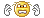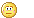We are here with you hands in hands to facilitate your learning & don't appreciate the idea of copying or replicating solutions. Read More>>

#www.vustudents.ning.com

 www.bit.ly/vucodes + Link For Assignments, GDBs & Online Quizzes Solution www.bit.ly/papersvu + Link For Past Papers, Solved MCQs, Short Notes & More

Dear Students! Share your Assignments / GDBs / Quizzes files as you receive in your LMS, So it can be discussed/solved timely. Add Discussion

# MTH603 - Numerical Analysis_GDB Due Date February 6,2013 at 00:00,February 7,2013 at 23:59.

Dear students, this is to inform that GDB will be started on February 6, 2013 at 00:00 and will be closed on February 7, 2013 at 23:59.

The topic for Graded Moderated Discussion Board is

"Compare the efficiency and characteristics of methods available to you for numerical integration"

+ How to Join Subject Study Groups & Get Helping Material?

+ How to become Top Reputation, Angels, Intellectual, Featured Members & Moderators?

+ VU Students Reserves The Right to Delete Your Profile, If?

Views: 553

.

+ http://bit.ly/vucodes (Link for Assignments, GDBs & Online Quizzes Solution)

+ http://bit.ly/papersvu (Link for Past Papers, Solved MCQs, Short Notes & More)

### Replies to This Discussionsallam to all vu fellows.......

plz share solution idea........

W aslam

Numerical ordinary differential equations is the part of numerical analysis which studies the numerical solution of ordinary differential equations (ODEs). This field is also known under the name numerical integration, but some people reserve this term for the computation of integrals.

Many differential equations cannot be solved analytically; however, in science and engineering, a numeric approximation to the solution is often good enough to solve a problem. The algorithms studied here can be used to compute such an approximation. An alternative method is to use techniques from calculus to obtain a series expansion of the solution.

Ordinary differential equations occur in many scientific disciplines, for instance in physics,chemistry, biology, and economics. In addition, some methods in numerical partial differential equations convert the partial differential equation into an ordinary differential equation, which must then be solved.

Numerical integration aims at approximating definite integrals using numerical techniques. There are many situations where numerical integration is needed. For example, several well defined functions do not have an anti-derivative, i.e. their anti-derivative cannot be expressed in terms of primitive function. A popular example is the function e��x2 whose anti-derivative does not exist. This function arises in a variety of applications such as those related to probability and statistics analyses. Furthermore, many applications in science and engineering are represented by integral differential equations that require a special treatment for the integral terms (e.g. expansion, liberalization, closure ...).Friends Humay in Points pr Disscussion Karni ........OK

NUMERICAL INTEGRATION (“Compare the efficiency and characteristics of methods available to you for numerical integration.”)

1. NEWTON-COTES INTEGRATION FORMULAE
2. THE TRAPEZOIDAL RULE ( COMPOSITE FORM)
3. SIMPSON’S RULES (COMPOSITE FORM)
4. ROMBERG’S INTEGRATION
5. DOUBLE INTEGRATION

NOW Go For It.............!>>>>>>>>>>>Keep LOving My Broken Heartimgimg /li>